# Circle calc: find a

Circle calc: find a
Go back to  'Circles'

Have you ever noticed the shape of a pizza?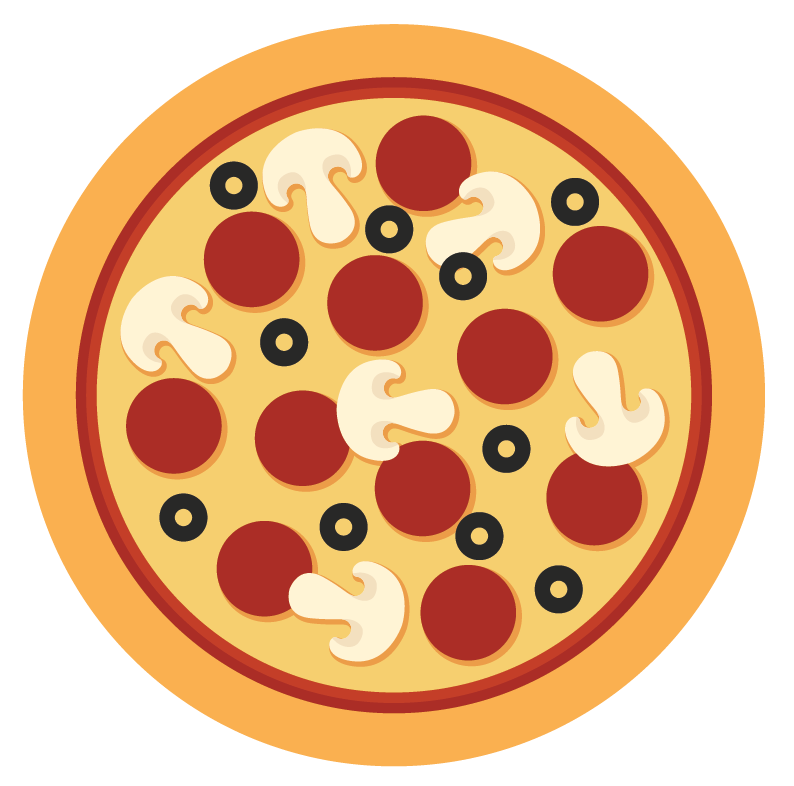What is this shape called?

You are right!

It is of circular shape.

Circles are one of the most commonly found shapes in the world. The fascinating properties of circles make it an important topic in geometry.

In this chapter, you will learn about circle diameter, area of a circle, circle calculator, circumference, and area of circles, in the concept of Circle. Check out the interactive simulation on the formation of a circle.

Try your hand at solving a few interesting practice questions at the end of the page.

## What is Circle?

A circle is formed by the set of points which are at a constant or fixed distance (radius) from a fixed point (center) in the plane.

The fixed point is called the origin or center of the circle.

The fixed distance of the points from the origin is called the radius.

### Shape of a Circle

Let's take a fixed point $$P$$ and try to draw lines from it.

Let's draw several lines of length 3 cm away from point $$P$$ in all possible directions.

When we join these ends together in a curve, it forms a circle.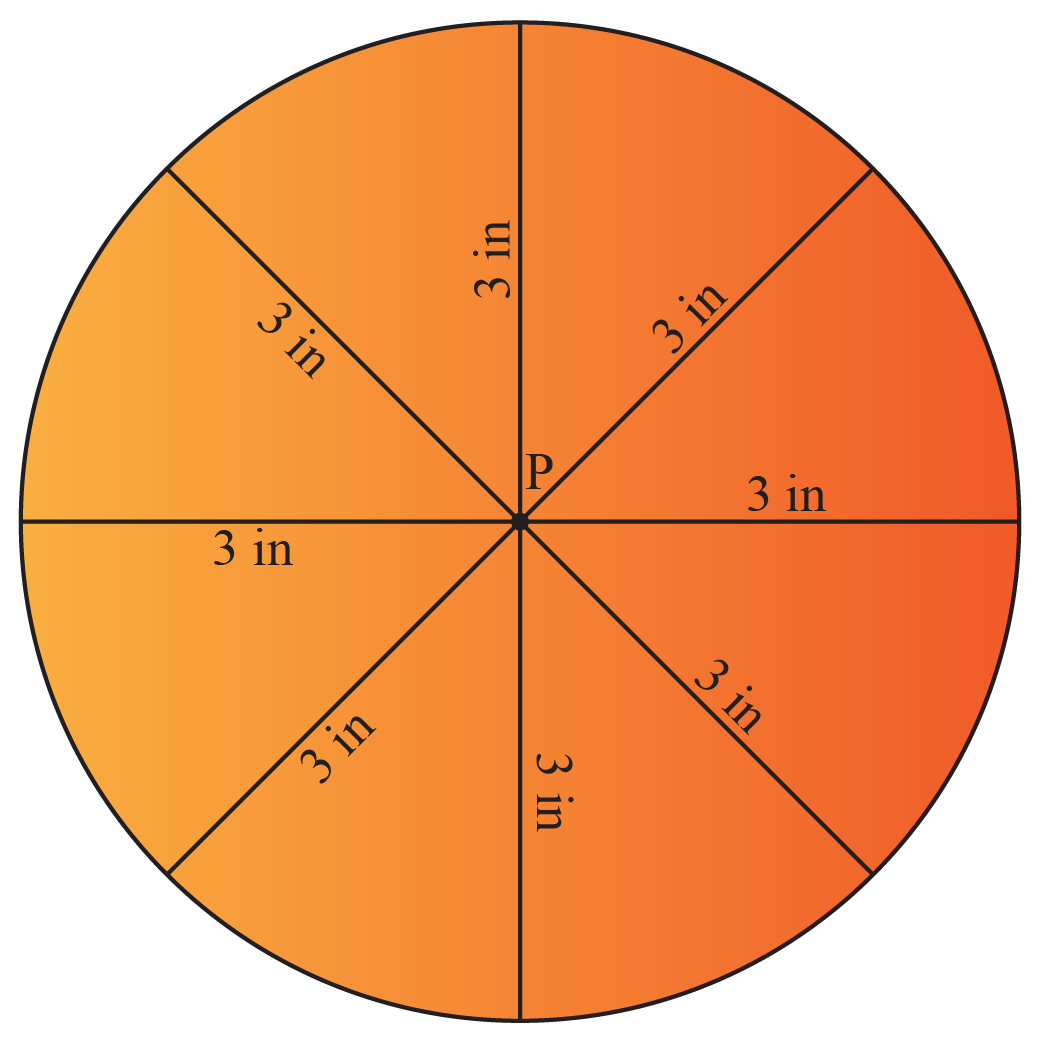Try this simulation which shows how the shape of a circle is formed by the collection of points which are equidistant from the center.

(Click on the buttons: Reset and See)

The circle we have drawn resembles the wheel of a cart.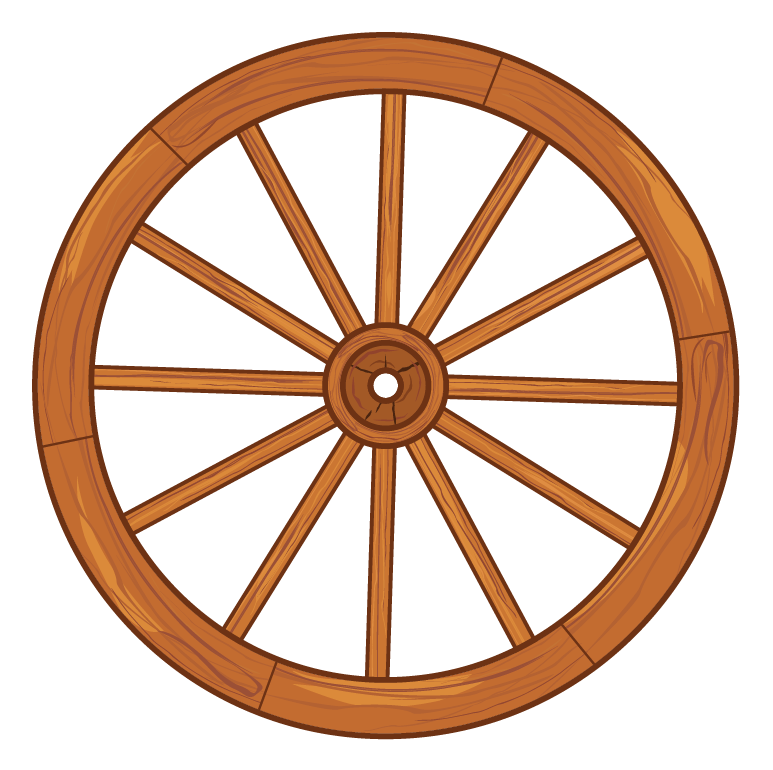Have you ever ridden a ferris wheel at a carnival?

Did you find anything common in these items?

Yes, it's their shape, which is a circle.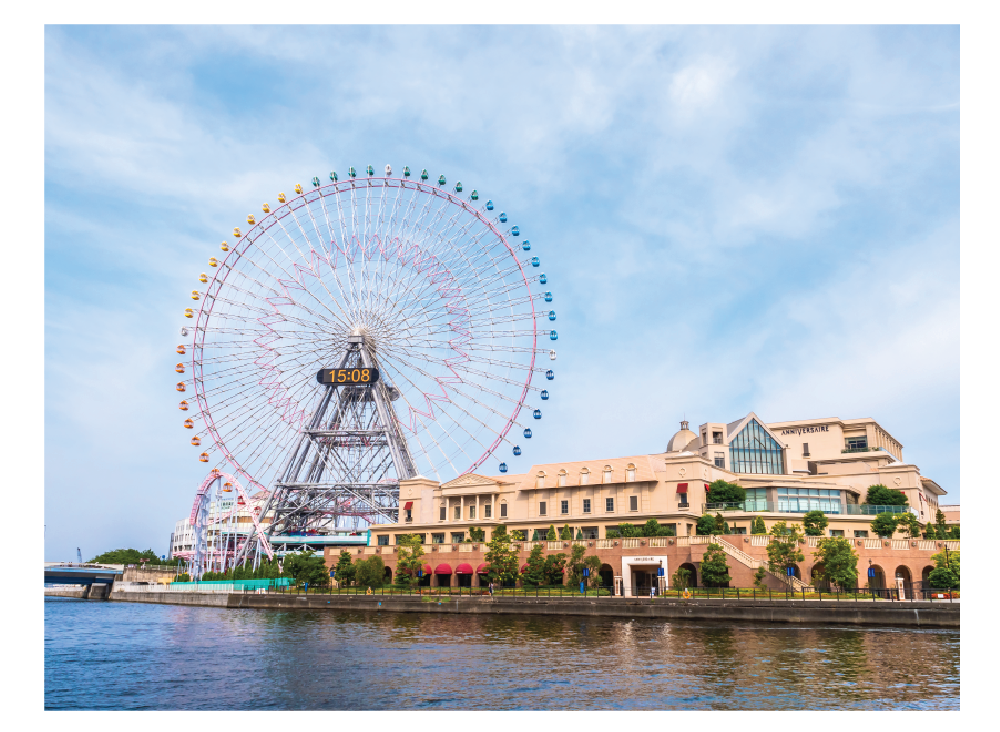## Properties of a Circle

The fascinating properties of circles makes it an important topic in math and geometry.

Imagine a circular park in your neighborhood.

Try to identify the various parts of a circle with the help of the figure and table given below.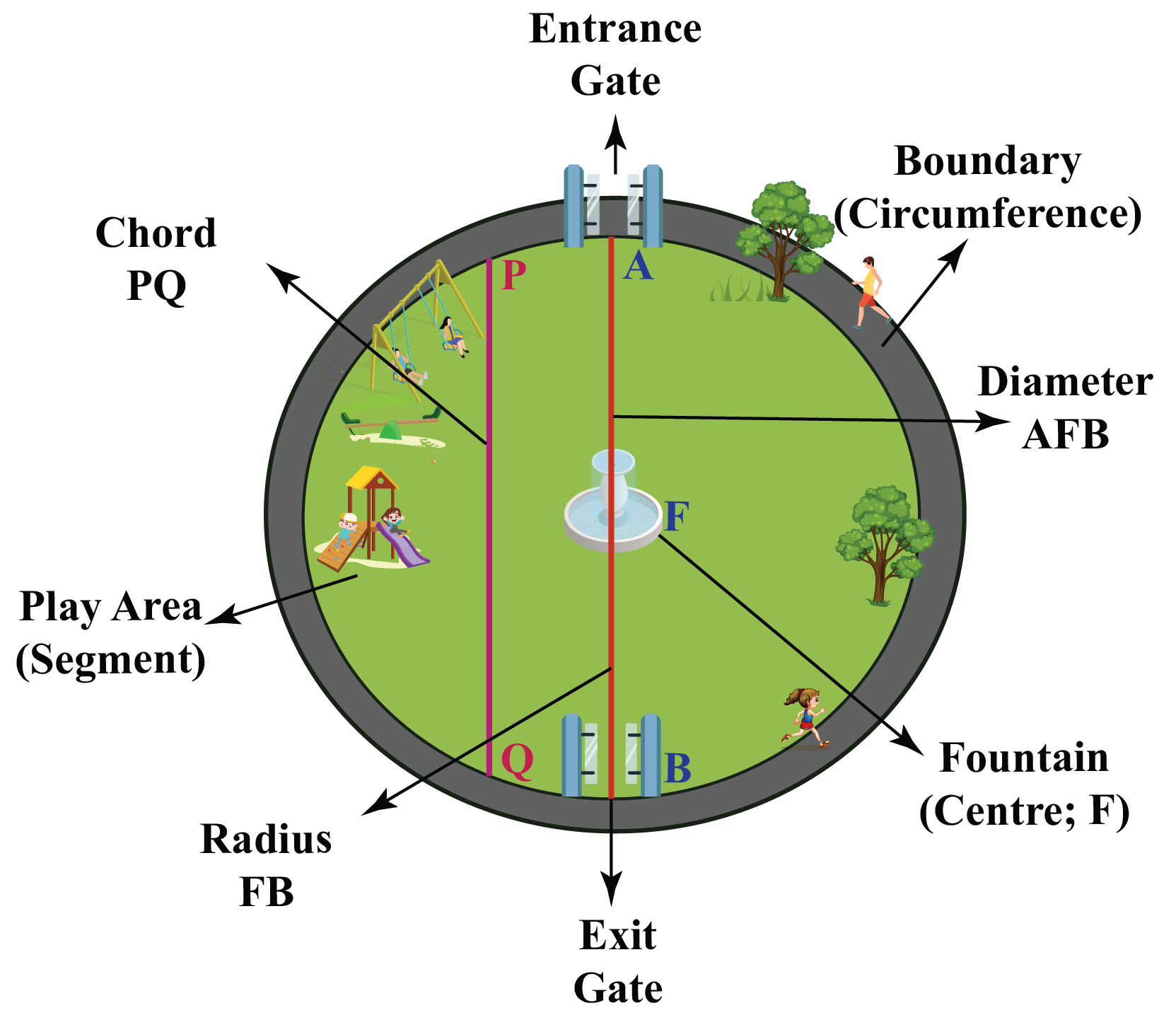In a Circle In our park Named by the letter
Centre Fountain $$F$$
Circumference Boundary
Chord Play area entrance $$PQ$$
Radius Distance from the fountain to the Entrance gate $$FA$$
Diameter Straight Line Distance between Entrance Gate and Exit Gate through the fountain $$AFB$$
Minor segment The smaller area of the park, which is shown as the Play area
Major segment The bigger area of the park, other than the Play area
Interior part of the circle The green area of the whole park
Exterior part of the circle The area outside the boundary of the park
Arc Any curved part on the circumference.

Could you find these parts in the park?

Well done!

## Parts of a Circle

### Radius

Radius is the distance from the center of a circle to the boundary of the circle.

Let's refer to the park that was shown earlier.

A circle can have multiple radii as they all start from the center and touch the boundary of the circle at various points.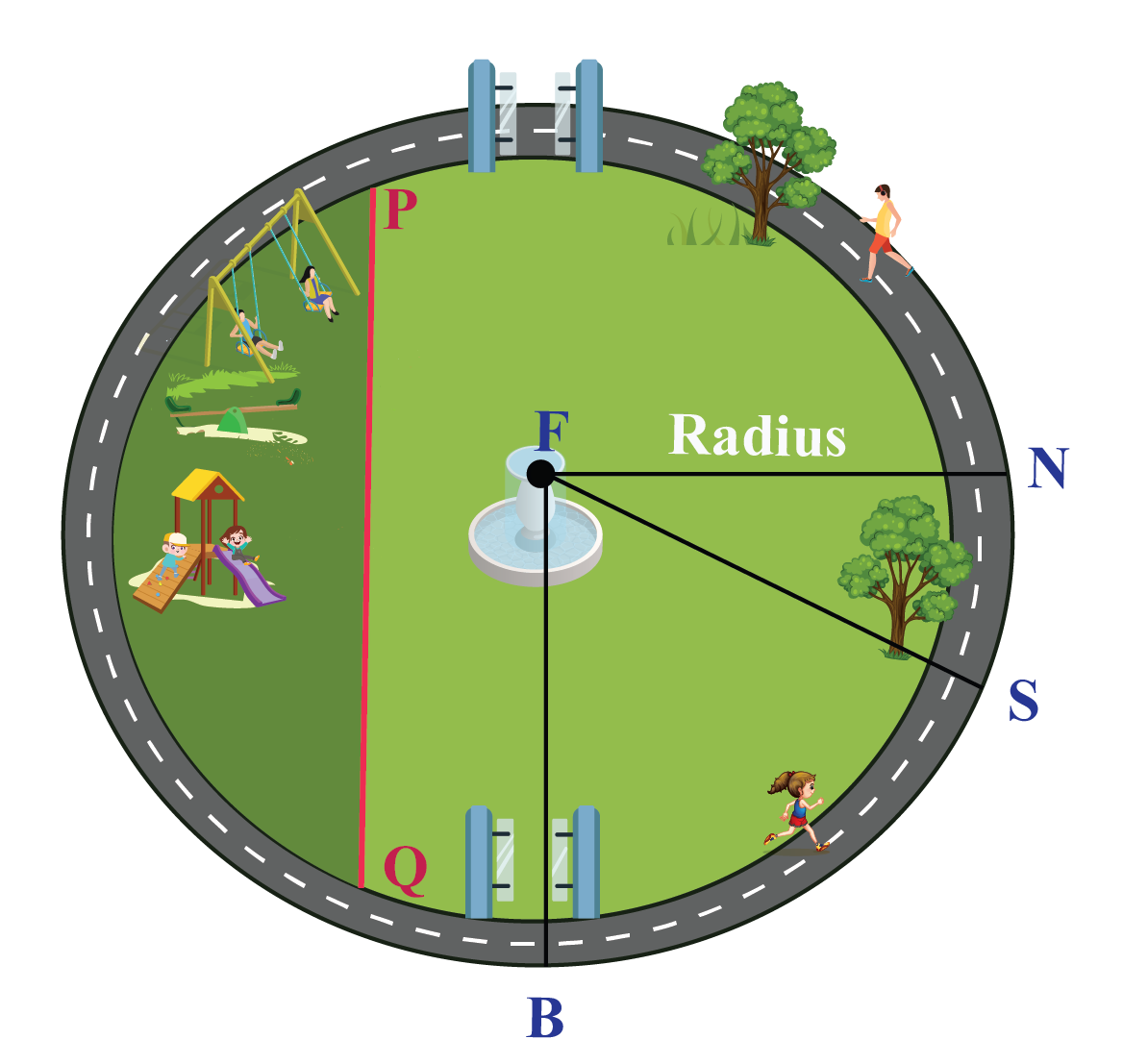### Diameter

Diameter is a straight line passing through the center that connects two points on the boundary of the circle.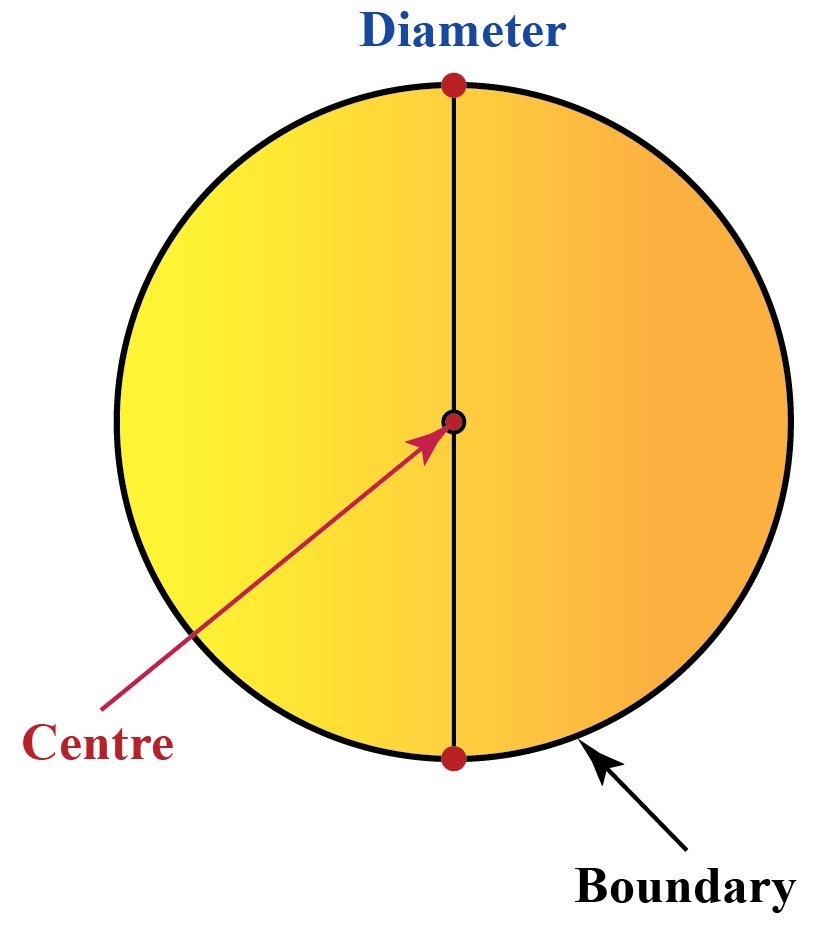Let's go back to the figure of the park.

We can see that $$AFB$$ is the diameter.

We should note that there can be multiple diameters in that park, but they should:

• pass through the fountain (center) in a straight line
• touch one boundary of the park to the other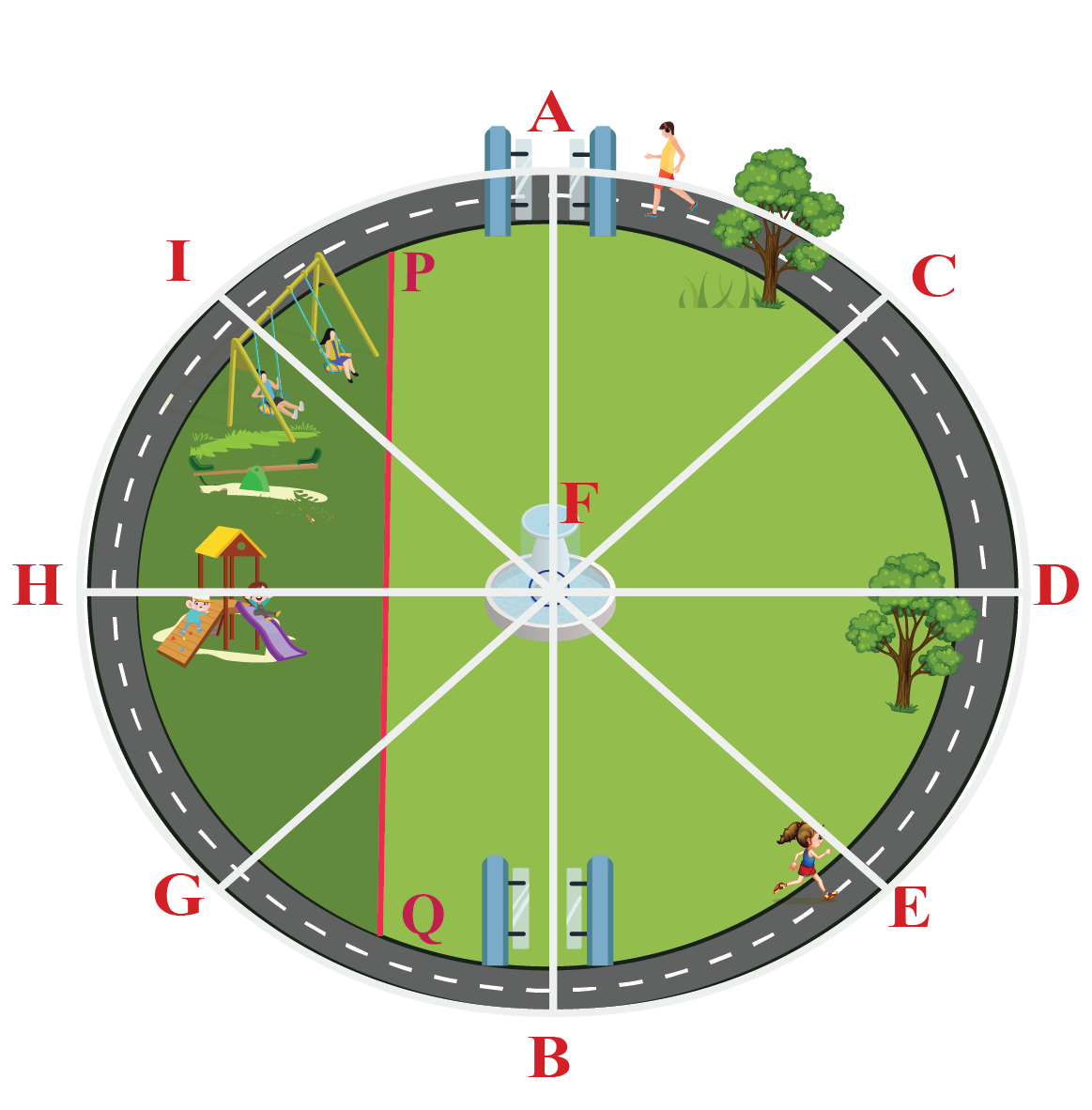### Chord

Any line segment that touches the circle at two different points is known as the chord of a circle.

Referring to the park, we can see that $$PQ$$ is the chord that shows where the play area starts.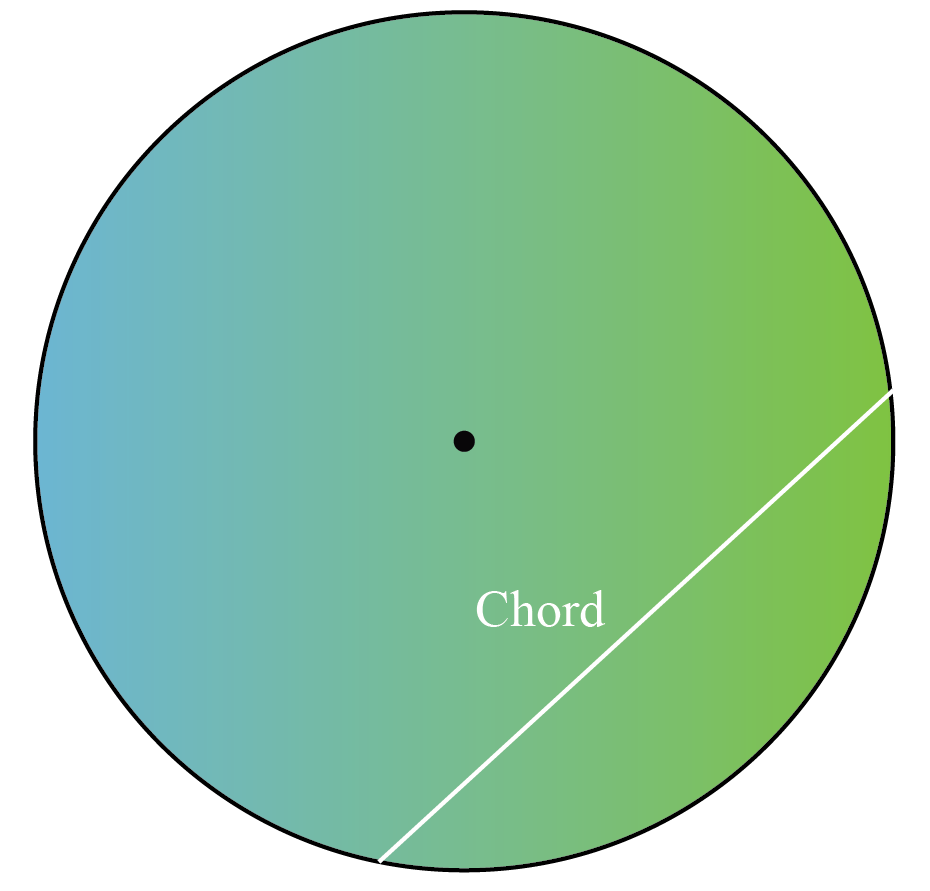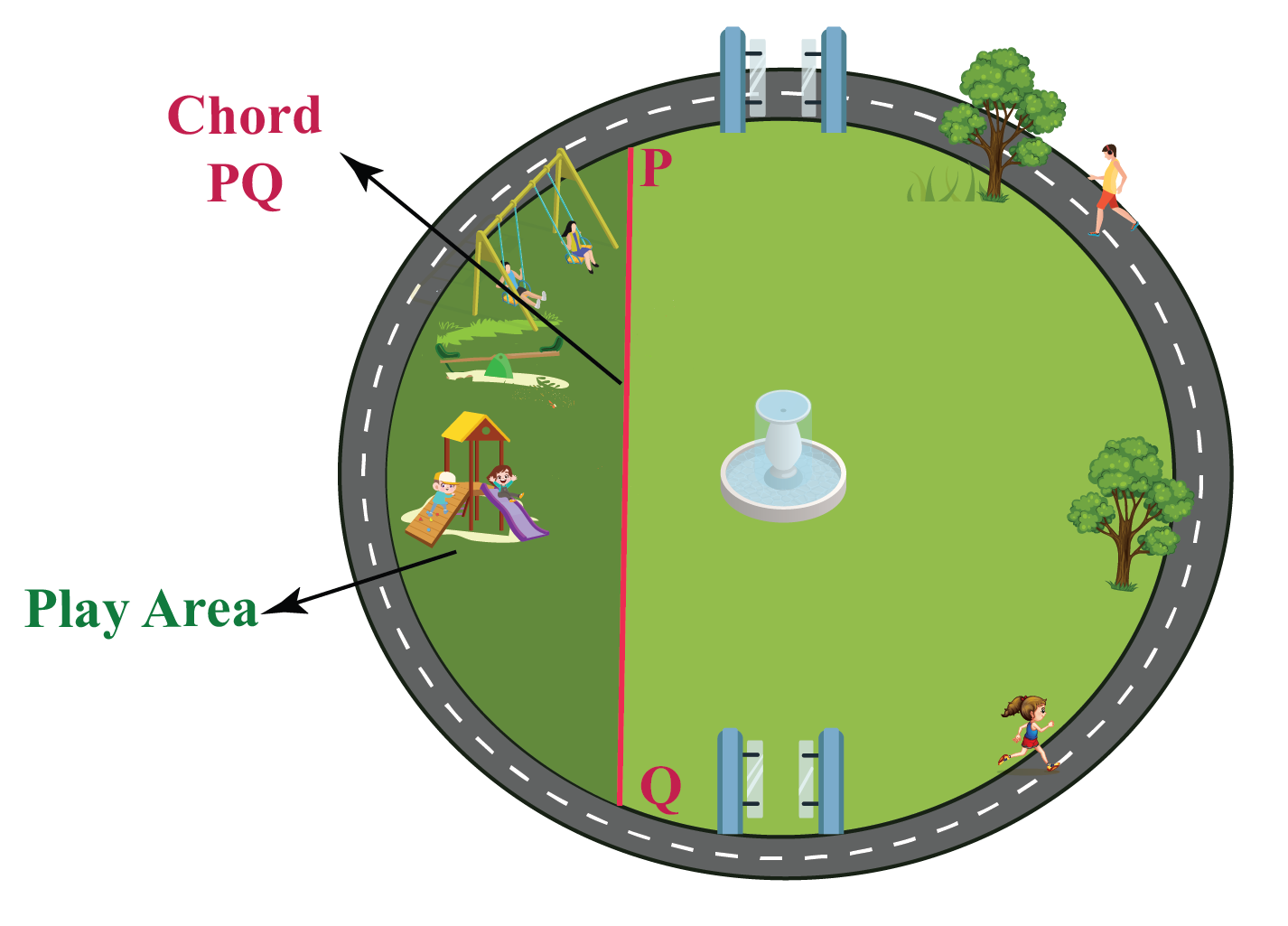### Circumference

The circumference of a circle is its boundary or the length of the complete arc of a circle.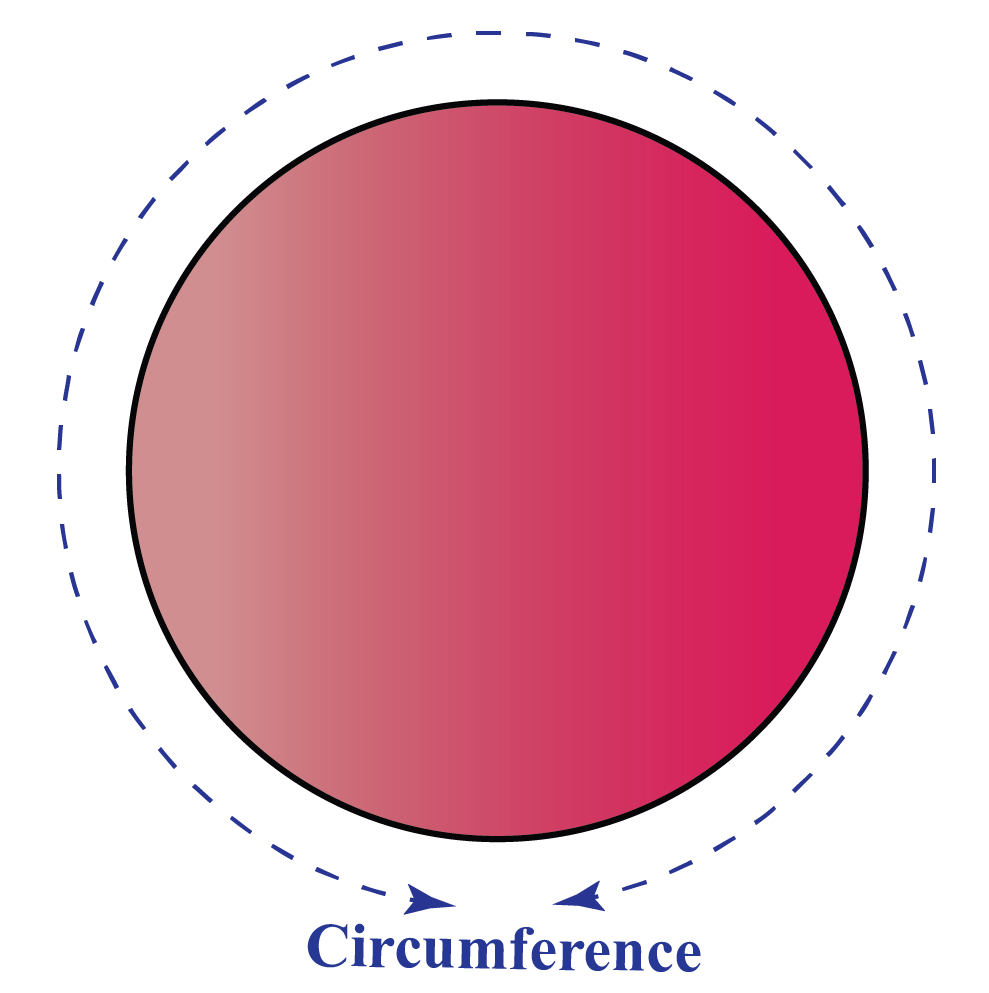Considering the same park, if you jog around the boundary of that park and complete one full circle without going anywhere else, that complete round is the circumference of a circle.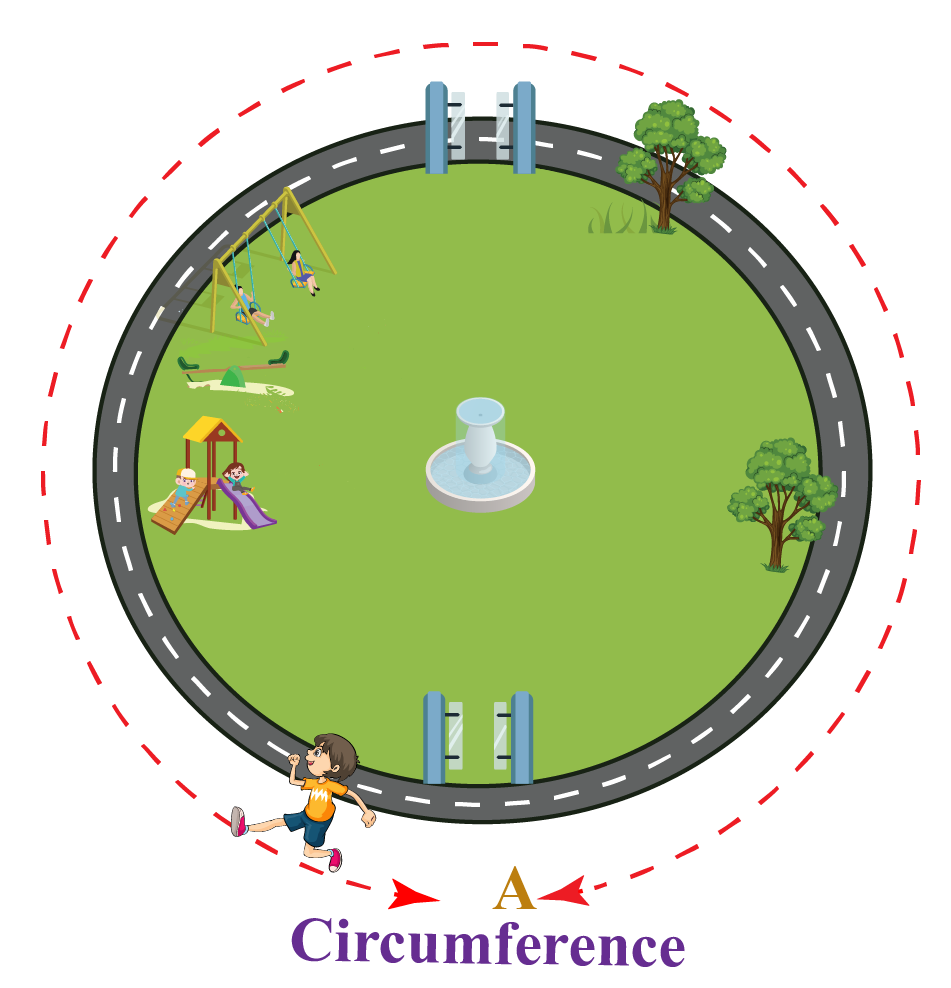More Important Topics
Numbers
Algebra
Geometry
Measurement
Money
Data
Trigonometry
Calculus